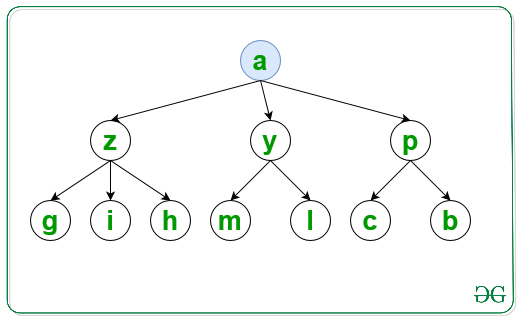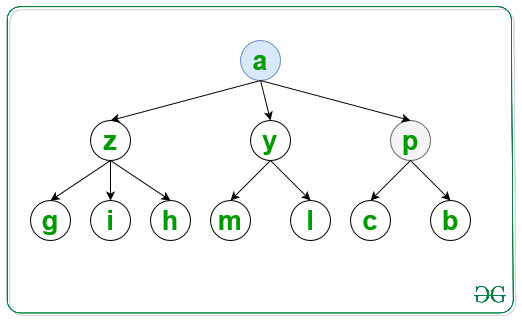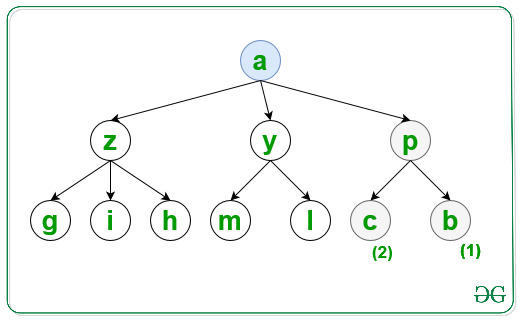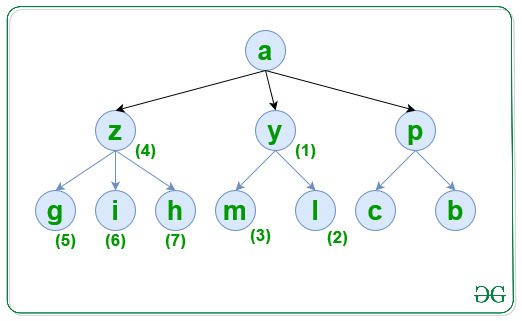Open in App
Not now

# Traverse graph in lexicographical order of nodes using DFS

• Last Updated : 15 Mar, 2023

Given a graph, G consisting of N nodes, a source S, and an array Edges[] of type {u, v} that denotes that there is an undirected edge between node u and v, the task is to traverse the graph in lexicographical order using DFS.

Examples:

Input: N = 10, M = 10, S = ‘a’, Edges[] = { { ‘a’, ‘y’ }, { ‘a’, ‘z’ }, { ‘a’, ‘p’ }, { ‘p’, ‘c’ }, { ‘p’, ‘b’ }, { ‘y’, ‘m’ }, { ‘y’, ‘l’ }, { ‘z’, ‘h’ }, { ‘z’, ‘g’ }, { ‘z’, ‘i’ } }
Output: a p b c y l m z g h i

Explanation:
For the first level visit the node and print it:Similarly visited the second level node p which is lexicographical smallest as:Similarly visited the third level for node p in lexicographical order as:Now the final traversal is shown in the below image and labelled as increasing order of number:Input: N = 6, S = ‘a’, Edges[] = { { ‘a’, ‘e’ }, { ‘a’, ‘d’ }, { ‘e’, ‘b’ }, { ‘e’, ‘c’ }, { ‘d’, ‘f’ }, { ‘d’, ‘g’ } }
Output: a d f g e b c

Approach: Follow the steps below to solve the problem:

• Initialize a map, say G to store all the adjacent nodes of a node according to lexicographical order of the nodes.
• Initialize a map, say vis to check if a node is already traversed or not.
• Traverse the Edges[] array and store all the adjacent nodes of each node of the graph in G.
• Finally, traverse the graph using DFS and print the visited nodes of the graph.

Below is the implementation of the above approach:

## C++

 `// C++ program  for the above approach` `#include ` `using` `namespace` `std;`   `// Function to traverse the graph in` `// lexicographical order using DFS` `void` `LexiDFS(map<``char``, set<``char``> >& G,` `             ``char` `S, map<``char``, ``bool``>& vis)` `{` `    ``// Mark S as visited nodes` `    ``vis[S] = ``true``;`   `    ``// Print value of visited nodes` `    ``cout << S << ``" "``;`   `    ``// Traverse all adjacent nodes of S` `    ``for` `(``auto` `i = G[S].begin();` `         ``i != G[S].end(); i++) {`   `        ``// If i is not visited` `        ``if` `(!vis[*i]) {`   `            ``// Traverse all the nodes` `            ``// which is connected to i` `            ``LexiDFS(G, *i, vis);` `        ``}` `    ``}` `}`   `// Utility Function to traverse graph` `// in lexicographical order of nodes` `void` `CreateGraph(``int` `N, ``int` `M, ``int` `S,` `                 ``char` `Edges[])` `{` `    ``// Store all the adjacent nodes` `    ``// of each node of a graph` `    ``map<``char``, set<``char``> > G;`   `    ``// Traverse Edges[] array` `    ``for` `(``int` `i = 0; i < M; i++) {`   `        ``// Add the edges` `        ``G[Edges[i]].insert(` `            ``Edges[i]);` `    ``}`   `    ``// Check if a node is already` `    ``// visited or not` `    ``map<``char``, ``bool``> vis;`   `    ``// Function Call` `    ``LexiDFS(G, S, vis);` `}`   `// Driver Code` `int` `main()` `{` `    ``int` `N = 10, M = 10, S = ``'a'``;` `    ``char` `Edges[M]` `        ``= { { ``'a'``, ``'y'` `}, { ``'a'``, ``'z'` `},` `            ``{ ``'a'``, ``'p'` `}, { ``'p'``, ``'c'` `}, ` `            ``{ ``'p'``, ``'b'` `}, { ``'y'``, ``'m'` `}, ` `            ``{ ``'y'``, ``'l'` `}, { ``'z'``, ``'h'` `}, ` `            ``{ ``'z'``, ``'g'` `}, { ``'z'``, ``'i'` `} };`   `    ``// Function Call` `    ``CreateGraph(N, M, S, Edges);`   `    ``return` `0;` `}`

## Java

 `// Java program for above approach` `import` `java.util.*;`   `class` `Graph{`   `// Function to traverse the graph in` `// lexicographical order using DFS` `static` `void` `LexiDFS(HashMap> G,` `            ``char` `S, HashMap vis)` `{` `    `  `    ``// Mark S as visited nodes` `    ``vis.put(S, ``true``);`   `    ``// Print value of visited nodes` `    ``System.out.print(S + ``" "``);`   `    ``// Traverse all adjacent nodes of S` `    ``if` `(G.containsKey(S))` `    ``{` `        ``for``(``char` `i : G.get(S)) ` `        ``{` `            `  `            ``// If i is not visited` `            ``if` `(!vis.containsKey(i) || !vis.get(i)) ` `            ``{` `                `  `                ``// Traverse all the nodes` `                ``// which is connected to i` `                ``LexiDFS(G, i, vis);` `            ``}` `        ``}` `    ``}` `}`   `// Utility Function to traverse graph` `// in lexicographical order of nodes` `static` `void` `CreateGraph(``int` `N, ``int` `M, ``char` `S,` `                        ``char``[][] Edges)` `{` `    `  `    ``// Store all the adjacent nodes` `    ``// of each node of a graph` `    ``HashMap> G = ``new` `HashMap<>();`   `    ``// Traverse Edges[] array` `    ``for``(``int` `i = ``0``; i < M; i++)` `    ``{` `        ``if` `(G.containsKey(Edges[i][``0``]))` `        ``{` `            ``Set temp = G.get(Edges[i][``0``]);` `            ``temp.add(Edges[i][``1``]);` `            ``G.put(Edges[i][``0``], temp);` `        ``}` `        ``else` `        ``{` `            ``Set temp = ``new` `HashSet<>();` `            ``temp.add(Edges[i][``1``]);` `            ``G.put(Edges[i][``0``], temp);` `        ``}` `    ``}` `    `  `    ``// Check if a node is already visited or not` `    ``HashMap vis = ``new` `HashMap<>();`   `    ``LexiDFS(G, S, vis);` `}`   `// Driver code` `public` `static` `void` `main(String[] args) ` `{` `    ``int` `N = ``10``, M = ``10``;` `    ``char` `S = ``'a'``;`   `    ``char``[][] Edges = { { ``'a'``, ``'y'` `}, { ``'a'``, ``'z'` `},` `                       ``{ ``'a'``, ``'p'` `}, { ``'p'``, ``'c'` `},` `                       ``{ ``'p'``, ``'b'` `}, { ``'y'``, ``'m'` `},` `                       ``{ ``'y'``, ``'l'` `}, { ``'z'``, ``'h'` `},` `                       ``{ ``'z'``, ``'g'` `}, { ``'z'``, ``'i'` `} };`   `    ``// Function Call` `    ``CreateGraph(N, M, S, Edges);` `}` `}`   `// This code is contributed by hritikrommie`

## Python3

 `# Python3 program  for the above approach` `G ``=` `[[] ``for` `i ``in` `range``(``300``)]` `vis ``=` `[``0` `for` `i ``in` `range``(``300``)]`   `# Function to traverse the graph in` `# lexicographical order using DFS` `def` `LexiDFS(S):` `    ``global` `G, vis` `    `  `    ``# Mark S as visited nodes` `    ``vis[``ord``(S)] ``=` `1`   `    ``# Prvalue of visited nodes` `    ``print` `(S,end``=``" "``)`   `    ``# Traverse all adjacent nodes of S` `    ``for` `i ``in` `G[``ord``(S)]:` `        ``# If i is not visited` `        ``if` `(``not` `vis[i]):` `            ``# Traverse all the nodes` `            ``# which is connected to i` `            ``LexiDFS(``chr``(i))`   `# Utility Function to traverse graph` `# in lexicographical order of nodes` `def` `CreateGraph(N, M, S, Edges):` `    ``global` `G` `    ``# Store all the adjacent nodes` `    ``# of each node of a graph`   `    ``# Traverse Edges[] array` `    ``for` `i ``in` `Edges:` `        ``# Add the edges` `        ``G[``ord``(i[``0``])].append(``ord``(i[``1``]))` `        ``G[``ord``(i[``0``])] ``=` `sorted``(G[``ord``(i[``0``])])`   `    ``# Function Call` `    ``LexiDFS(S)`   `# Driver Code` `if` `__name__ ``=``=` `'__main__'``:` `    ``N ``=` `10` `    ``M ``=` `10` `    ``S ``=` `'a'` `    ``Edges``=``[ [``'a'``, ``'y'` `],[ ``'a'``, ``'z'` `],` `           ``[ ``'a'``, ``'p'` `],[ ``'p'``, ``'c'` `],` `           ``[ ``'p'``, ``'b'` `],[ ``'y'``, ``'m'` `],` `           ``[ ``'y'``, ``'l'` `],[ ``'z'``, ``'h'` `],` `           ``[ ``'z'``, ``'g'` `],[ ``'z'``, ``'i'` `] ]`   `    ``# Function Call` `    ``CreateGraph(N, M, S, Edges);`   `# This code is contributed by mohitkumar29.`

## Javascript

 ``

## C#

 `// C# program  for the above approach` `using` `System;` `using` `System.Collections.Generic;`   `class` `Graph {` `    ``// Function to traverse the graph in` `    ``// lexicographical order using DFS` `    ``static` `void` `LexiDFS(List<``int``>[] G, ``int` `S, ``bool``[] vis) {` `        ``// Mark S as visited nodes` `        ``vis[S] = ``true``;`   `        ``// Print value of visited nodes` `        ``Console.Write(Convert.ToChar(S) + ``" "``);`   `        ``// Traverse all adjacent nodes of S` `        ``foreach` `(``int` `i ``in` `G[S]) {` `            ``// If i is not visited` `            ``if` `(!vis[i]) {` `                ``// Traverse all the nodes` `                ``// which is connected to i` `                ``LexiDFS(G, i, vis);` `            ``}` `        ``}` `    ``}`   `    ``// Utility Function to traverse graph` `    ``// in lexicographical order of nodes` `    ``static` `void` `CreateGraph(``int` `N, ``int` `M, ``char` `S, ``char``[][] Edges) {` `        ``// Store all the adjacent nodes` `        ``// of each node of a graph` `        ``List<``int``>[] G = ``new` `List<``int``>;` `        ``for` `(``int` `i = 0; i < G.Length; i++) {` `            ``G[i] = ``new` `List<``int``>();` `        ``}`   `        ``// Traverse Edges[] array` `        ``for` `(``int` `i = 0; i < M; i++) {` `            ``// Add the edges` `            ``G[Edges[i]].Add(Edges[i]);`   `            ``G[Edges[i]].Sort();` `        ``}`   `        ``// Check if a node is already visited or not` `        ``bool``[] vis = ``new` `bool``;`   `        ``LexiDFS(G, S, vis);` `    ``}`   `    ``// Driver code` `    ``static` `void` `Main(``string``[] args) {` `        ``int` `N = 10;` `        ``int` `M = 10;` `        ``char` `S = ``'a'``;`   `        ``char``[][] Edges = { ` `            ``new` `char``[] { ``'a'``, ``'y'` `}, ` `            ``new` `char``[] { ``'a'``, ``'z'` `},` `            ``new` `char``[] { ``'a'``, ``'p'` `}, ` `            ``new` `char``[] { ``'p'``, ``'c'` `},` `            ``new` `char``[] { ``'p'``, ``'b'` `}, ` `            ``new` `char``[] { ``'y'``, ``'m'` `},` `            ``new` `char``[] { ``'y'``, ``'l'` `}, ` `            ``new` `char``[] { ``'z'``, ``'h'` `},` `            ``new` `char``[] { ``'z'``, ``'g'` `}, ` `            ``new` `char``[] { ``'z'``, ``'i'` `}` `        ``};`   `        ``// Function Call` `        ``CreateGraph(N, M, S, Edges);` `    ``}` `}`   `// This code is contributed by Jay`

Output:

`a p b c y l m z g h i`

Time Complexity: O(N * log(N))
Auxiliary Space: O(N)

My Personal Notes arrow_drop_up
Related Articles